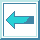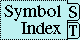#System Class FLOAT

Class Precedence List:

Description:

A float is a mathematical rational (but not a Common Lisp rational) of the form s*f*b^e-p, where s is +1 or -1, the sign; b is an integer greater than 1, the base or radix of the representation; p is a positive integer, the precision (in base-b digits) of the float; f is a positive integer between b^p-1 and b^p-1 (inclusive), the significand; and e is an integer, the exponent. The value of p and the range of e depends on the implementation and on the type of float within that implementation. In addition, there is a floating-point zero; depending on the implementation, there can also be a ``minus zero''. If there is no minus zero, then 0.0 and -0.0 are both interpreted as simply a floating-point zero. (= 0.0 -0.0) is always true. If there is a minus zero, (eql -0.0 0.0) is false, otherwise it is true.

The types short-float, single-float, double-float, and long-float are subtypes of type float. Any two of them must be either disjoint types or the same type; if the same type, then any other types between them in the above ordering must also be the same type. For example, if the type single-float and the type long-float are the same type, then the type double-float must be the same type also.

Compound Type Specifier Kind:

Abbreviating.

Compound Type Specifier Syntax:

float [lower-limit [upper-limit]]

Compound Type Specifier Arguments:

lower-limit, upper-limit---interval designators for type float. The defaults for each of lower-limit and upper-limit is the symbol *.

Compound Type Specifier Description:

This denotes the floats on the interval described by lower-limit and upper-limit.